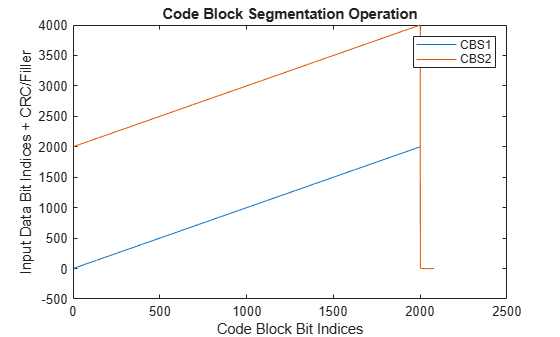# nrCodeBlockSegmentLDPC

LDPC code block segmentation and CRC attachment

Since R2018b

## Syntax

``cbs = nrCodeBlockSegmentLDPC(blk,bgn)``

## Description

example

````cbs = nrCodeBlockSegmentLDPC(blk,bgn)` splits the input data block `blk` into code block segments based on the base graph number `bgn`, as specified in TS 38.212 Section 5.2.2 . The function appends cyclic redundancy check (CRC) and filler bits to each code block segment in `cbs` (if applicable). `nrCodeBlockSegmentLDPC` provides input to low-density parity-check (LDPC) coders in transport channels, including downlink and uplink shared channels, and paging channels.```

## Examples

collapse all

Create a random sequence of binary input data.

`in = randi([0,1],4000,1);`

Perform LDPC code block segmentation.

```cbs1 = nrCodeBlockSegmentLDPC(in,1); cbs2 = nrCodeBlockSegmentLDPC(in,2);```

When the base graph number is 1, the segmentation results in one code block segment. When the base graph number is 2, the segmentation results in two code block segments. Segmentation occurs only if the input length is greater than the maximum code block size. The maximum code block size is 8448 when the base graph number is 1 and 3840 when the base graph number is 2.

`size(cbs1)`
```ans = 1×2 4224 1 ```
`size(cbs2)`
```ans = 1×2 2080 2 ```

Create a ramp data input and perform code block segmentation.

`cbs = nrCodeBlockSegmentLDPC([1:4000]',2);`

The input of length 4000 is split into two code block segments of equal size with 24B CRC and filler bits attached. To see how the input maps onto the output, plot the input data indices relative to the corresponding code block segment indices.

```plot(cbs) legend('CBS1','CBS2') xlabel('Code Block Bit Indices'); ylabel('Input Data Bit Indices + CRC/Filler'); title('Code Block Segmentation Operation')```## Input Arguments

collapse all

Input data block, specified as a column vector of real numbers.

Data Types: `double` | `int8` | `logical`

Base graph number, specified as `1` or `2`.

Data Types: `double`

## Output Arguments

collapse all

Code block segments, returned as an integer or real matrix. Each column corresponds to a separate code block segment. The number of code block segments depends on the maximum code block size of the LDPC coder, `Kcb`, and the length of the input `blk`, `B`. If `bgn` is set to `1`, `Kcb` = 8448. If `bgn` is set to `2`, `Kcb` = 3840. If `B``Kcb`, then the function does not perform segmentation and does not append CRC to the resulting code block. If `B` > `Kcb`, the segmentation results in several smaller code blocks with a type-24B CRC bits appended.

The function appends filler bits to each code block (with or without CRC) if necessary. The filler bits ensure that the code block segments entering the LDPC coder have a valid length and are a multiple of the LDPC lifting size. To accommodate the filler bits represented by –1, the data type of `cbs` is cast to `int8` when the input `blk` is logical. Otherwise, `cbs` inherits the data type of the input `blk`.

Data Types: `double` | `int8`

 3GPP TS 38.212. “NR; Multiplexing and channel coding.” 3rd Generation Partnership Project; Technical Specification Group Radio Access Network.